# RS Aggarwal Class 7 Solutions Chapter 4 Rational Numbers Ex 4G

## RS Aggarwal Class 7 Solutions Chapter 4 Rational Numbers Ex 4G

These Solutions are part of RS Aggarwal Solutions Class 7. Here we have given RS Aggarwal Solutions Class 7 Chapter 4 Rational Numbers Ex 4G.

Other Exercises

OBJECTIVE QUESTIONS
Mark (✓) against the correct answer in each of the following:
Question 1.
Solution:
(b)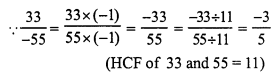Question 2.
Solution:
(b)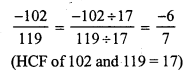Question 3.
Solution:
(a)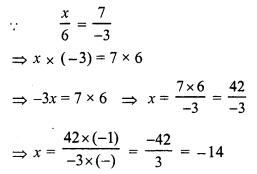Question 4.
Solution:
(c)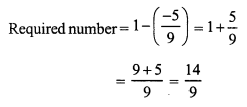Question 5.
Solution:
(b)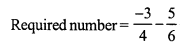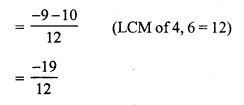Question 6.
Solution:
(a)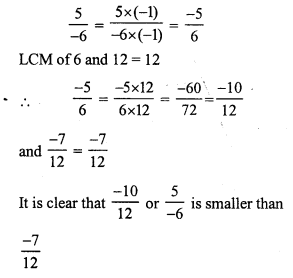Question 7.
Solution:
(a)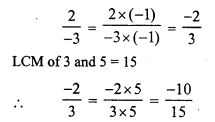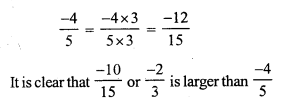Question 8.
Solution:
(c)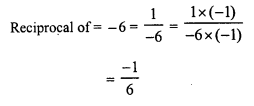Question 9.
Solution:
(b)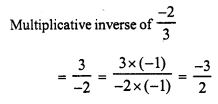Question 10.
Solution: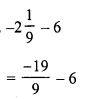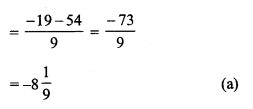Question 11.
Solution: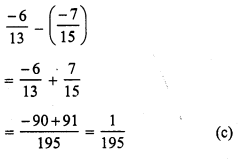Question 12.
Solution: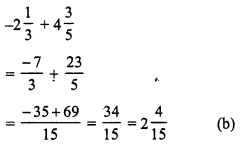Question 13.
Solution: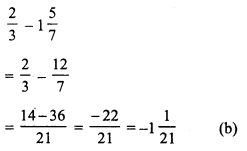Question 14.
Solution: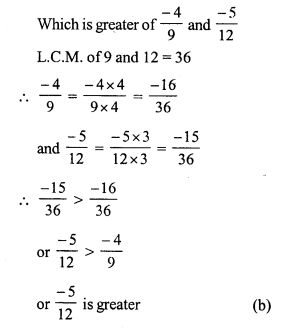Question 15.
Solution: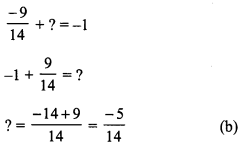Question 16.
Solution: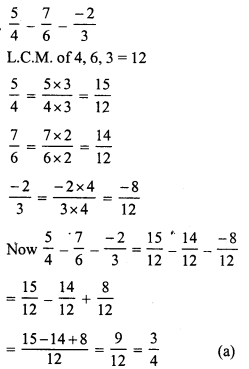Question 17.
Solution: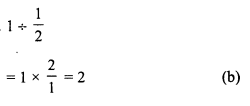Question 18.
Solution: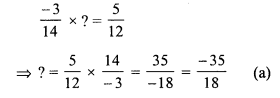Question 19.
Solution: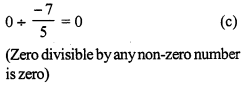Question 20.
Solution: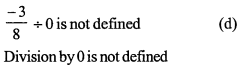Hope given RS Aggarwal Solutions Class 7 Chapter 4 Rational Numbers Ex 4G are helpful to complete your math homework.

If you have any doubts, please comment below. Learn Insta try to provide online math tutoring for you.